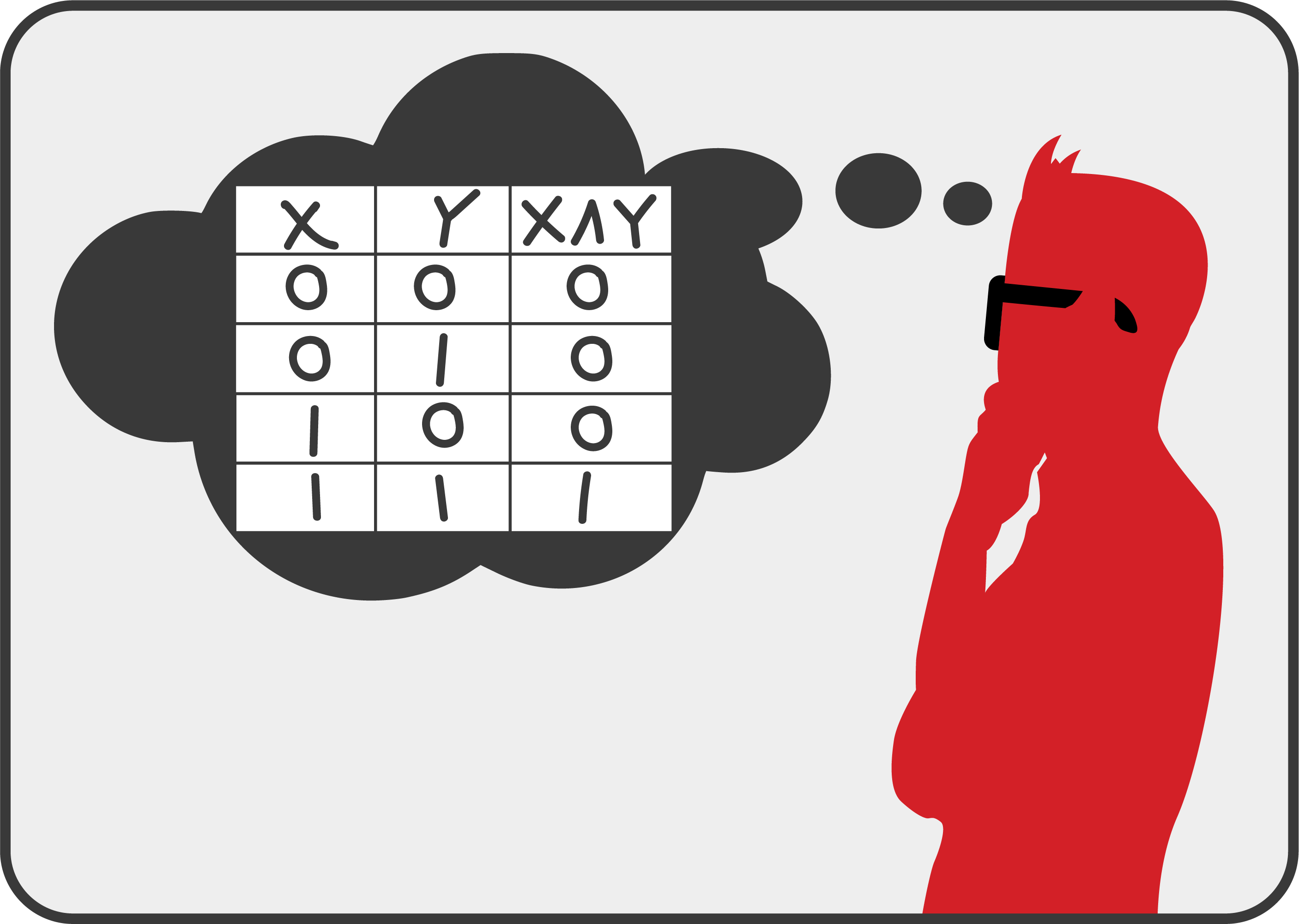01 October, 2020

## Boolean Math (AND Logic) - CISSP Domain 3Author: Bill McCauley
Share:

To begin with, Boolean math is used to define the rules on how computer systems evaluate data. Because Boolean is based on a binary system, it only has two values, 1 and 0. When evaluating these values, a one (1) is going to be equal to a True condition and a zero (0) is going to be equal to a False condition. Basically 1/0 is the same as on/off or true/false.

Next, since we’re specifically looking at the AND logic here, we need to define what this is and how it works. The AND operation is defined by checking two values to see if they are true. In other words, if both X and Y are True (or equal to 1), then the result is also going to be True (1). It’s also important to note that an AND operation is represented by using the ∧ symbol, called an up-caret. There are different types of Boolean logic that can be applied, but when you see a Boolean math problem with this symbol, then you’ll know to apply the AND logic.

Below is what is known as a truth table. These are used to map out the different combinations and results of our binary values. Again, because binary only has 2 values, it is easy to map out all the different combinations of 0 and 1.

Since there is a possibility of seeing a Boolean mathematics problem on your CISSP exam, we’re going to walk through an example problem and apply the Boolean AND logic to solve the problem.

Example Boolean Problem:

X:           0 1 1 0 0 1 0 1
Y:            1 0 1 0 0 1 1 0
___________________
X ∧ Y:

The first thing that you will want to do is to verify what type of logic to apply to the problem. Because we see the up-caret symbol, , we know to use the AND logic here.

We’re going to compare between the two sets of values in X and Y. In this example, we have one set of values for X (0 1 1 0 0 1 0 1) and another set of values for Y (1 0 1 0 0 1 1 0). In order to solve the problem, we’ll start on the far left and use our truth table to evaluate the X and Y values in the first column.

Using the AND logic, if the values for both X and Y are 1, then the results will be 1. Otherwise, the values will be 0, or False. Therefore, when looking at this first column, we ask the question, “Are both X and Y True (1)?”. However, since X and Y are not both equal to 1, then the result of comparing these two values is 0.

So, when we work down each column in this problem and apply the AND logic, then the final answer should look like this:

You can easily practice this by making a random set of digits (0 or 1) for X and Y, and then solve them using the AND operational logic. Hopefully this has been helpful for anyone struggling with Boolean math or wanting to prepare for any Boolean math problems that might show up on the CISSP exam.

This has been a quick look at the AND Boolean logic.  There is an audio/video version of this material here, for anyone who prefers that format.  If you’re interested in security fundamentals, we have a Professionally Evil Fundamentals (PEF) channel that covers a variety of technology topics.  We also answer general basic questions in our Knowledge Center.  Finally, if you’re looking for a penetration test, training for your organization, or just have general security questions please Contact Us.